## Quiz 26 : Capital Investment AnalysisLooking for Accounting Homework Help?# Quiz 26 : Capital Investment Analysis

The capital budgeting decision process is a complete multifaceted and analytical process. Capital budgeting analysis estimates, such as the cash flows, discount rate, time period and total investment amount. This results of analysis should be used to either support or reject a project, capital budgeting should not be used to justify an assumed net present value. The analyst should not work backward, filling assumed numbers that will produce the desired net present value. Such a reverse approach reduces the credibility of the entire process. In the given case, Danielle who is new analyst analysis the purchasing of warehouse according to standards and reject the construction because purchase of warehouse does not add much value to business and does not increase sales as a result of warehouse. But Jerrod who is plant manager argued the Danielle that warehouse is needed to store the production and if we can store more goods, then we will have more sales. On the basis of above information, the contention of Jerrod is not correct because purchasing of warehouses does not guaranty to increase sales. Hence Danielle is advised t not to consider the Jerrod plea.

The average rate of return has the following disadvantages: The principal objections to the use of the average rate of return method are its failure to consider the expected cash flows from the proposals and the timing of these flows.

The average rate of return is the net incremental return from the new investment opportunity. In this method, the calculation is done on the generally accepted accounting principles. The formula to calculate the average rate of return is average incremental increase in annual net income should be divided by the (cost of original investment plus residual value/2). The calculation of average net income for Testing Equipment is shown below: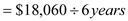The average net annual income is \$3,010. The average investment for testing equipment is calculated below: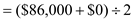The average investment is \$43,000. The Average rate of return for testing equipment can be calculated as follows: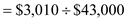The expected average rate of return from testing equipment is 0.07 or 7%. The calculation of average net income for vehicle is shown below: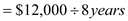The average net annual income is \$1,500. The average investment for vehicle is calculated below: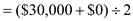The average investment is \$15,000. The Average rate of return for vehicle can be calculated as follows: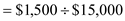The expected average rate of return from vehicle is 0.1 or 10%.